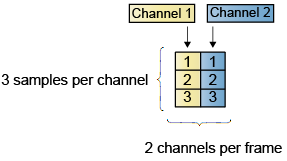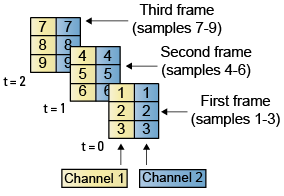Main Content

## Create Signals for Frame-Based Processing

In frame-based processing, blocks process data one frame at a time. Each frame of data contains sequential samples from an independent channel. Each channel is represented by a column of the input signal. For example, from a frame-based processing perspective, the following 3-by-2 matrix has two channels, each of which contains three samples.When you configure a block to perform frame-based processing, the block interprets an M-by-1 vector as a single-channel signal containing M samples per frame. Similarly, the block interprets an M-by-N matrix as a multichannel signal with N independent channels and M samples per channel. For example, in frame-based processing, blocks interpret the following sequence of 3-by-2 matrices as a two-channel signal with a frame size of 3.Using frame-based processing is advantageous for many signal processing applications because you can process multiple samples at once. By buffering your data into frames and processing multisample frames of data, you can often improve the computational time of your signal processing algorithms. To perform frame-based processing, you must have a DSP System Toolbox™ license.

For more information about the recent changes to frame-based processing, see the Frame-based processing changes section of the DSP System Toolbox Release Notes.

This page discusses creating signals for frame-based processing using the Sine Wave block and the Signal From Workspace block. Note that the block receiving this signal implements sample-based processing or frame-based processing on the signal based on the parameters set in the block dialog box.

### Create Signals Using Sine Wave Block

1. Create a new Simulink® model.

2. From the Sources library, click-and-drag a Sine Wave block into the model.

3. From the Matrix Operations library, click-and-drag a Matrix Sum block into the model.

4. From the Simulink Sinks library, click-and-drag a To Workspace block into the model.

5. Connect the blocks in the order in which you added them to your model.

6. Double-click the Sine Wave block, and set the block parameters as follows:

• Amplitude = ```[1 3 2]```

• Frequency = ```[100 250 500]```

• Sample time = `1/5000`

• Samples per frame = `64`

Based on these parameters, the Sine Wave block outputs three sinusoids with amplitudes `1`, `3`, and `2` and frequencies `100`, `250`, and `500` Hz, respectively. The sample period, 1/5000, is 10 times the highest sinusoid frequency, which satisfies the Nyquist criterion. The frame size is 64 for all sinusoids, and, therefore, the output has 64 rows.

7. Save these parameters and close the dialog box by clicking .

You have now successfully created a three-channel signal, with `64` samples per each frame, using the Sine Wave block. The rest of this procedure describes how to add these three sinusoids together.

8. Double-click the Matrix Sum block. Set the Sum over parameter to ```Specified dimension```, and set the Dimension parameter to `2`. Click .

9. In the Debug tab of the model toolstrip, select > .

10. Run the model.

Your model should now look similar to the following figure. You can also open the model by typing `ex_usingsinwaveblkfb` at the MATLAB® command line.The three signals are summed point-by-point by a Matrix Sum block. Then, they are exported to the MATLAB workspace.

11. At the MATLAB command line, type `plot(yout(1:100))`.

Your plot should look similar to the following figure.This figure represents a portion of the sum of the three sinusoids. You have now added the channels of a three-channel signal together and displayed the results in a figure window.

### Create Signals Using Signal From Workspace Block

Frame-based processing can significantly improve the performance of your model by decreasing the amount of time it takes your simulation to run. This topic describes how to create a two-channel signal with a sample period of 1 second, a frame period of 4 seconds, and a frame size of 4 samples using the Signal From Workspace block.

1. Create a new Simulink model.

2. From the Sources library, click-and-drag a Signal From Workspace block into the model.

3. From the Simulink Sinks library, click-and-drag a To Workspace block into the model.

4. Connect the two blocks.

5. Double-click the Signal From Workspace block, and set the block parameters as follows.

• Signal = ```[1:10; 1 1 0 0 1 1 0 0 1 1]'```

• Sample time = `1`

• Samples per frame = `4`

• Form output after final data value by = `Setting to zero`

Based on these parameters, the Signal From Workspace block outputs a two-channel signal with a sample period of 1 second, a frame period of 4 seconds, and a frame size of four samples. After the block outputs the signal, all subsequent outputs have a value of zero. The two channels contain the following values:

• Channel 1: 1, 2, 3, 4, 5, 6, 7, 8, 9, 10, 0, 0,...

• Channel 2: 1, 1, 0, 0, 1, 1, 0, 0, 1, 1, 0, 0,...

6. Save these parameters and close the dialog box by clicking .

7. In the Debug tab of the model toolstrip, select > .

8. Run the model.

The following figure is a graphical representation of the model's behavior during simulation. You can also open the model by typing `ex_usingsfwblkfb` at the MATLAB command line.9. At the MATLAB command line, type `yout`.

The following is the output displayed at the MATLAB command line.

```yout = 1 1 2 1 3 0 4 0 5 1 6 1 7 0 8 0 9 1 10 1 0 0 0 0 ```

Note that zeros were appended to the end of each channel. You have now successfully created a two-channel signal and exported it to the MATLAB workspace.

Watch now